# Seymour, Paul D.

Author(s): Harvey; Reed; Seymour; Wood

Conjecture   For every graph,
(a)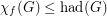(b)(c)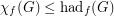.

Keywords: fractional coloring, minors

## Seymour's r-graph conjecture ★★★

Author(s): Seymour

An-graph is an-regular graphwith the property that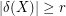for everywith odd size.

Conjecturefor every-graph.

Keywords: edge-coloring; r-graph

## Non-edges vs. feedback edge sets in digraphs ★★★

Author(s): Chudnovsky; Seymour; Sullivan

For any simple digraph, we letbe the number of unordered pairs of nonadjacent vertices (i.e. the number of non-edges), andbe the size of the smallest feedback edge set.

Conjecture  Ifis a simple digraph without directed cycles of length, then.

Keywords: acyclic; digraph; feedback edge set; triangle free

## Seagull problem ★★★

Author(s): Seymour

Conjecture   Everyvertex graph with no independent set of sizehas a complete graph on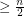vertices as a minor.

Keywords: coloring; complete graph; minor

## Seymour's Second Neighbourhood Conjecture ★★★

Author(s): Seymour

Conjecture   Any oriented graph has a vertex whose outdegree is at most its second outdegree.

Keywords: Caccetta-Häggkvist; neighbourhood; second; Seymour

## Bases of many weights ★★★

Author(s): Schrijver; Seymour

Letbe an (additive) abelian group, and for everylet.

Conjecture   Letbe a matroid on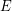, let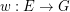be a map, put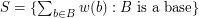and. Then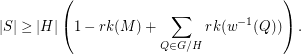Keywords: matroid; sumset; zero sum

## Alon-Saks-Seymour Conjecture ★★★

Author(s): Alon; Saks; Seymour

Conjecture   Ifis a simple graph which can be written as an union ofedge-disjoint complete bipartite graphs, then.

## Seymour's self-minor conjecture ★★★

Author(s): Seymour

Conjecture   Every infinite graph is a proper minor of itself.

Keywords: infinite graph; minor

## Faithful cycle covers ★★★

Author(s): Seymour

Conjecture   Ifis a graph,is admissable, and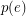is even for every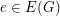, thenhas a faithful cover.

Keywords: cover; cycle

## Cycle double cover conjecture ★★★★

Author(s): Seymour; Szekeres

Conjecture   For every graph with no bridge, there is a list of cycles so that every edge is contained in exactly two.

Keywords: cover; cycle